# What Is L’Hopital’s Rule? (5 Key Concepts You Should Grasp)

In calculus and analysis, we often need to evaluate limits – sometimes, these limits have indeterminate forms.  L’Hopital’s Rule can help us to evaluate some of these limits – but it can only be applied in certain cases.

So, what is L’Hopital’s Rule?  L’Hopital’s Rule is used to evaluate limits that have indeterminate forms, such as 0/0 or ∞/∞. L’Hopital’s Rule says that if f(x) and g(x) are differentiable with limx->c[f(x)/g(x)] indeterminate, then limx->c[f(x)/g(x)] = limx->c[f’(x)/g’(x)] [f’(x) and g’(x) are the derivatives of f(x) and g(x)].

Of course, L’Hopital’s Rule cannot be applied in some cases – for example, when g’(x) is zero, we cannot use this rule.

In this article, we’ll talk about L’Hopital’s Rule, what it is, and when it can be applied.  We’ll also look at some examples to show exactly how to use this rule to help evaluate limits with an indeterminate form.

Let’s get started.

## What Is L’Hopital’s Rule?

L’Hopital’s Rule helps us to find the value of some limits that have an indeterminate form, such as 0/0 or ∞/∞.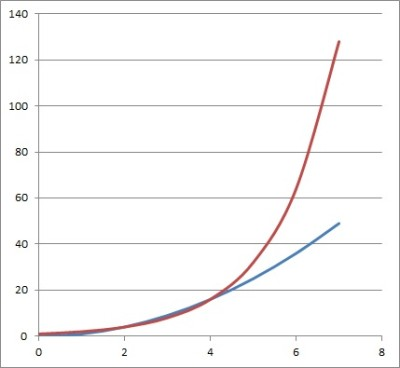L’Hopital’s Rule helps us to compare how quickly two functions approach zero or infinity. This helps us to resolve and evaluate some limits that have an indeterminate form, such as 0/0.

If f(x) and g(x) are differentiable functions and the limit limx->c[f(x)/g(x)] is 0/0 or ∞/∞ (an indeterminate form), then L’Hopital’s Rule says that:

• limx->c[f(x)/g(x)] = limx->c[f’(x)/g’(x)]

where f’(x) is the derivative function of f(x) and g’(x) is the derivative function of g(x).

### Why Does L’Hopital’s Rule Work?

L’Hopital’s Rule works because it helps us to compare how fast two “competing” functions approach zero or infinity.

L’Hopital’s Rule allows us to compare the growth rates of two functions (that is, f’(x) and g’(x)), rather than the functions themselves (f(x) and g(x)).

In other words, we are looking at the slopes of the functions instead of the functions themselves.

Note that we can continue this process repeatedly: if one application of L’Hopital’s Rule still yields an indeterminate form, we can use the rule again (provided that the derivative functions f’(x) and g’(x) are still differentiable).

However, keep in mind that there are some restrictions as far as when you can use L’Hopital’s Rule.

### When Can You Use L’Hopital’s Rule?

To use L’Hopital’s Rule, we must have an indeterminate form when we try to find limx->c[f(x)/g(x)].  That is, the result of this limit is 0/0 or ∞/∞.

Also, the functions f(x) and g(x) must also be differentiable (on an open interval).  Note that a differentiable function is also continuous.

In addition, the denominator g’(x) must be nonzero (on an open interval) to avoid a zero denominator in the quotient limx->c[f’(x)/g’(x)].

Finally, the limit limx->c[f’(x)/g’(x)] must exist.

If any of these conditions are broken, then we cannot use L’Hopital’s Rule.

### Does L’Hopital’s Rule Apply To Limits At Infinity?

L’Hopital’s Rule does apply to limits at infinity, as long as the four conditions above are satisfied.  We can make the change of variables t = 1/x to change a limit as x approaches infinity to a limit as t approaches zero:

• limx->∞[f(x)/g(x)] = limt->0[f(1/t)/g(1/t)]

### Can L’Hopital’s Rule Be Applied To Every Limit?

L’Hopital’s Rule cannot be applied to every limit.  As mentioned earlier, there are several conditions to use this rule, including the condition that the limit of the quotient must be an indeterminate form.

For example, if the limit of the quotient limx->c[f(x)/g(x)] is 0/4, or 4/0, or 1/2, etc., then we cannot use L’Hopital’s Rule (since 0/4, 4/0, and 1/2 are not indeterminate forms).

### Can You Use L’Hopital’s Rule For 1/0?

You cannot use L’Hopital’s Rule for 1/0.  The reason is that a limit of 1/0 is not an indeterminate form.

Likewise, you cannot use L’Hopital’s Rule for 2/0, or 3/0, or 4/0, etc., since none of these are indeterminate forms.

### How To Use L’Hopital’s Rule

To use L’Hopital’s Rule, there are a few basic steps:

• 1. Make sure that limx->c[f(x)/g(x)] is an indeterminate form (either 0/0 or ∞/∞).
• 2. Make sure that f(x) and g(x) are differentiable.
• 3. Find the derivatives f’(x) and g’(x) with respect to the variable x.
• 4. Make sure that g’(x) is nonzero.
• 5. Take the limit limx->c[f’(x)/g’(x)], simplifying if necessary.
• 6. Apply L’Hopital’s Rule again, if necessary.

Let’s take a look at some examples to see how it works.

#### Example 1: How To Use L’Hopital’s Rule (0/0)

Let’s say that we want to evaluate the following limit:

• limx->0[(3x)/(ex – 1)]

When we substitute x = 0 into the quotient, we get:

• 3(0) / (e0 – 1) = 0 / (1 – 1) = 0/0

This is an indeterminate form, so we can use L’Hopital’s Rule with f(x) = 3x and g(x) = ex – 1.

Note that both f(x) and g(x) are differentiable functions, with f’(x) = 3 and g’(x) = ex.

Also, g’(x) is nonzero (since there is no value of x such that ex = 0, because ln(0) is undefined).

So, by L’Hopital’s Rule, we have:

• limx->c[f(x)/g(x)] = limx->c[f’(x)/g’(x)]
• limx->0[(3x)/(ex – 1)] = limx->0[(3)/(ex)]
• limx->0[(3x)/(ex – 1)] = 3/(e0)
• limx->0[(3x)/(ex – 1)] = 3/1
• limx->0[(3x)/(ex – 1)] = 3

So, the limit evaluates to a value of 3.

You can see a visual of the two graphs f(x) and g(x) below.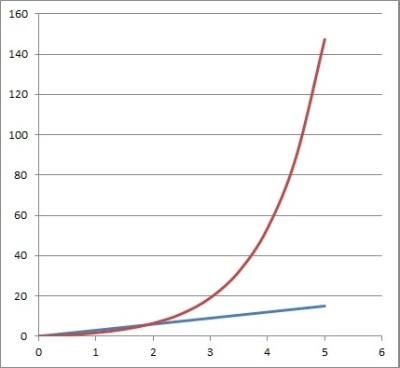This graph shows f(x) = 3x (blue line) and g(x) = ex – 1 (red curve). Since g(x) grows faster, the limit of f(x) over g(x) is zero as x approaches zero.

#### Example 2: How To Use L’Hopital’s Rule (0/0)

Let’s say that we want to evaluate the following limit:

• limx->0[(4x2)/(e2x – ex)]

When we substitute x = 0 into the quotient, we get:

• 4(02) / (e2*0 – e0) = 0 / (1 – 1) = 0/0

This is an indeterminate form, so we can use L’Hopital’s Rule with f(x) = 4x2 and g(x) = e2x – ex.

Note that both f(x) and g(x) are differentiable functions, with f’(x) = 8x and g’(x) = 2e2x – ex.

Also, g’(x) is nonzero near x = 0, since:

• 2e2x – ex = 0
• ex(2ex – 1) = 0

which means g’(x) = 0 only when x = ln(1/2).

So, by L’Hopital’s Rule, we have:

• limx->c[f(x)/g(x)] = limx->c[f’(x)/g’(x)]
• limx->0[(4x2)/(e2x – ex)] = limx->0[(8x)/(2e2x – ex)]
• limx->0[(4x2)/(e2x – ex)] = [(8(0))/(2e2(0) – e0)]
• limx->0[(4x2)/(e2x – ex)] = [0/(2(1) – 1)]
• limx->0[(4x2)/(e2x – ex)] = [0/(1)]
• limx->0[(4x2)/(e2x – ex)] = 0

So, the limit evaluates to a value of 0.

You can see a visual of the two graphs f(x) and g(x) below.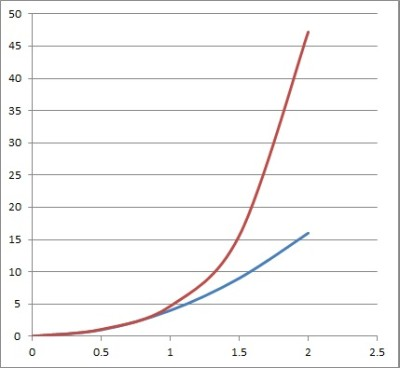This graph shows f(x) = 4x2 (blue curve) and g(x) = e2x – ex. (red curve). Since g(x) grows faster, the limit of f(x) over g(x) is zero as x approaches zero.

#### Example 3: How To Use L’Hopital’s Rule (infinity/infinity)

Let’s say that we want to evaluate the following limit:

• limx->∞[5x/ex]

When we substitute x = ∞ into the quotient, we get:

• 5(∞)/e = ∞/∞

This is an indeterminate form, so we can use L’Hopital’s Rule with f(x) = 5x and g(x) = ex.

Note that both f(x) and g(x) are differentiable functions, with f’(x) = 5 and g’(x) = ex.

Also, g’(x) is nonzero (since there is no value of x such that ex = 0, because ln(0) is undefined).

So, by L’Hopital’s Rule, we have:

• limx->c[f(x)/g(x)] = limx->c[f’(x)/g’(x)]
• limx->∞[5x/ex] = limx->∞[5/ex]
• limx->∞[5x/ex] = 5/e
• limx->∞[5x/ex] = 5/∞
• limx->∞[5x/ex] = 0

So, the limit evaluates to a value of 0.

You can see a visual of the two graphs f(x) and g(x) below.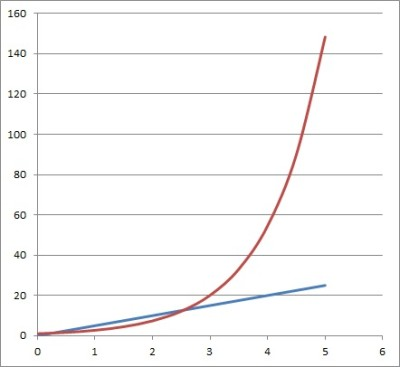This graph shows f(x) = 5x (blue line) and g(x) = ex (red curve). Since g(x) grows faster, the limit of f(x) over g(x) is zero as x approaches infinity.

#### Example 4: How To Use L’Hopital’s Rule (infinity/infinity)

Let’s say that we want to evaluate the following limit:

• limx->∞[x2/ex]

When we substitute x = ∞ into the quotient, we get:

• (2)/e = ∞/∞

This is an indeterminate form, so we can use L’Hopital’s Rule with f(x) = x2 and g(x) = ex.

Note that both f(x) and g(x) are differentiable functions, with f’(x) = 2x and g’(x) = ex.

Also, g’(x) is nonzero (since there is no value of x such that ex = 0, because ln(0) is undefined).

So, by L’Hopital’s Rule, we have:

• limx->c[f(x)/g(x)] = limx->c[f’(x)/g’(x)]
• limx->∞[x2/ex] = limx->∞[2x/ex]
• limx->∞[x2/ex] = 2(∞)/e
• limx->∞[x2/ex] = ∞/∞

Since this limit is still an indeterminate form, we will apply L’Hopital’s Rule a second time, with f’(x) = 2x and g’(x) = ex.

Note that both f’(x) and g’(x) are differentiable functions, with f’’(x) = 2 and g’’(x) = ex.

Also, g’’(x) is nonzero (since there is no value of x such that ex = 0, because ln(0) is undefined).

So, by L’Hopital’s Rule, we have:

• limx->c[f’(x)/g’(x)] = limx->c[f’’(x)/g’’(x)]
• limx->∞[2x/ex] = limx->∞[2/ex]
• limx->∞[2x/ex] = 2/e
• limx->∞[2x/ex] = 2/∞
• limx->∞[2x/ex] = 0

So, the limit evaluates to a value of 0.

You can see a visual of the two graphs f(x) and g(x) below.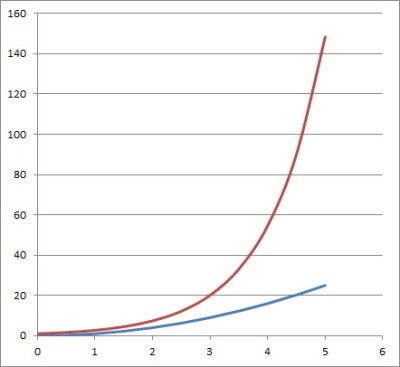This graph shows f(x) = x2 (blue curve) and g(x) = ex. (red curve). Since g(x) grows faster, the limit of f(x) over g(x) is zero as x approaches infinity.

## Conclusion

Now you know what L’Hopital’s Rule is, when to use it, and why it works.  You also know to apply it and when it cannot be applied.

You can find a helpful video on the factoring technique for indeterminate forms on this page from Problem Solved Math.